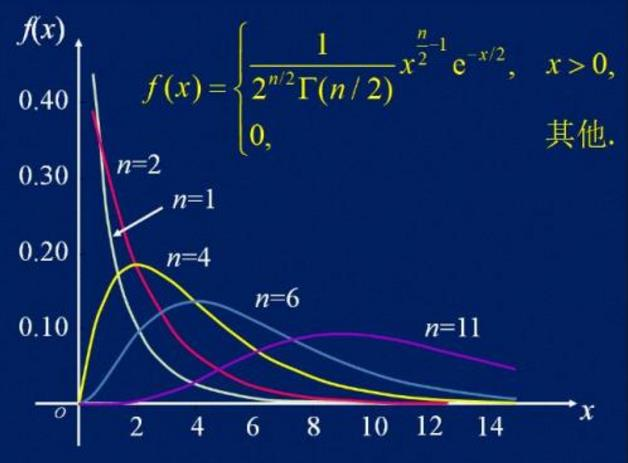### 卡方分布$DF = (Row-1) \times (Column - 1)$

### 期望值计算公式

$E= {M_r \times M_c \over Total}$

### 卡方计算

$\chi^2= \Sigma_r \Sigma_c {O_{rc}^2-E_{rc}^2 \over E_rc}$

### 何时使用Yates校正卡方值

$Yates's \chi^2= \Sigma_r \Sigma_c {|O_{rc}^2-E_{rc}^2| -0.5 \over E_rc}$

| —- |男|女|
|有效|30|20|
|无效|40|15|

### 赞助